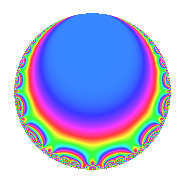# Properties

 Label 1800.2.b.aLevel 1800 Weight 2 Character orbit 1800.b Analytic conductor 14.373 Analytic rank 0 Dimension 2 CM No Inner twists 2

# Related objects

## Newspace parameters

 Level: $$N$$ = $$1800 = 2^{3} \cdot 3^{2} \cdot 5^{2}$$ Weight: $$k$$ = $$2$$ Character orbit: $$[\chi]$$ = 1800.b (of order $$2$$ and degree $$1$$)

## Newform invariants

 Self dual: No Analytic conductor: $$14.3730723638$$ Analytic rank: $$0$$ Dimension: $$2$$ Coefficient field: $$\Q(\sqrt{-2})$$ Coefficient ring: $$\Z[a_1, a_2]$$ Coefficient ring index: $$1$$ Sato-Tate group: $\mathrm{SU}(2)[C_{2}]$

## $q$-expansion

Coefficients of the $$q$$-expansion are expressed in terms of $$\beta = \sqrt{-2}$$. We also show the integral $$q$$-expansion of the trace form.

 $$f(q)$$ $$=$$ $$q + \beta q^{2} -2 q^{4} + 3 \beta q^{7} -2 \beta q^{8} +O(q^{10})$$ $$q + \beta q^{2} -2 q^{4} + 3 \beta q^{7} -2 \beta q^{8} + \beta q^{11} + 3 \beta q^{13} -6 q^{14} + 4 q^{16} + 2 \beta q^{17} -4 q^{19} -2 q^{22} + 6 q^{23} -6 q^{26} -6 \beta q^{28} + 6 q^{29} -6 \beta q^{31} + 4 \beta q^{32} -4 q^{34} -3 \beta q^{37} -4 \beta q^{38} + 7 \beta q^{41} -8 q^{43} -2 \beta q^{44} + 6 \beta q^{46} -11 q^{49} -6 \beta q^{52} -12 q^{53} + 12 q^{56} + 6 \beta q^{58} + \beta q^{59} + 6 \beta q^{61} + 12 q^{62} -8 q^{64} -8 q^{67} -4 \beta q^{68} -14 q^{73} + 6 q^{74} + 8 q^{76} -6 q^{77} -6 \beta q^{79} -14 q^{82} + 2 \beta q^{83} -8 \beta q^{86} + 4 q^{88} -5 \beta q^{89} -18 q^{91} -12 q^{92} + 10 q^{97} -11 \beta q^{98} +O(q^{100})$$ $$\operatorname{Tr}(f)(q)$$ $$=$$ $$2q - 4q^{4} + O(q^{10})$$ $$2q - 4q^{4} - 12q^{14} + 8q^{16} - 8q^{19} - 4q^{22} + 12q^{23} - 12q^{26} + 12q^{29} - 8q^{34} - 16q^{43} - 22q^{49} - 24q^{53} + 24q^{56} + 24q^{62} - 16q^{64} - 16q^{67} - 28q^{73} + 12q^{74} + 16q^{76} - 12q^{77} - 28q^{82} + 8q^{88} - 36q^{91} - 24q^{92} + 20q^{97} + O(q^{100})$$

## Character Values

We give the values of $$\chi$$ on generators for $$\left(\mathbb{Z}/1800\mathbb{Z}\right)^\times$$.

 $$n$$ $$577$$ $$901$$ $$1001$$ $$1351$$ $$\chi(n)$$ $$1$$ $$-1$$ $$-1$$ $$-1$$

## Embeddings

For each embedding $$\iota_m$$ of the coefficient field, the values $$\iota_m(a_n)$$ are shown below.

For more information on an embedded modular form you can click on its label.

Label $$\iota_m(\nu)$$ $$a_{2}$$ $$a_{3}$$ $$a_{4}$$ $$a_{5}$$ $$a_{6}$$ $$a_{7}$$ $$a_{8}$$ $$a_{9}$$ $$a_{10}$$
251.1
 − 1.41421i 1.41421i
1.41421i 0 −2.00000 0 0 4.24264i 2.82843i 0 0
251.2 1.41421i 0 −2.00000 0 0 4.24264i 2.82843i 0 0
 $$n$$: e.g. 2-40 or 990-1000 Significant digits: Format: Complex embeddings Normalized embeddings Satake parameters Satake angles

## Inner twists

Char. orbit Parity Mult. Self Twist Proved
1.a Even 1 trivial yes
24.f Even 1 yes

## Hecke kernels

This newform can be constructed as the intersection of the kernels of the following linear operators acting on $$S_{2}^{\mathrm{new}}(1800, [\chi])$$:

 $$T_{7}^{2} + 18$$ $$T_{23} - 6$$ $$T_{43} + 8$$